GeeksforGeeks App
Open AppBrowser
Continue

# How to Prepare Data Before Deploying a Machine Learning Model?

Before deploying a machine learning model, it is important to prepare the data to ensure that it is in the correct format and that any errors or inconsistencies have been cleaned. Here are some steps to prepare data before deploying a machine learning model:

1. Data collection: Collect the data that you will use to train your model. This could be from a variety of sources such as databases, CSV files, or APIs.
2. Data cleaning: Check for any missing, duplicate or inconsistent data and clean it. This may include removing any irrelevant columns, filling in missing values, and formatting data correctly.
3. Data exploration: Explore the data to gain insights into its distribution, relationships between features, and any outliers. Use visualization tools to help identify patterns, anomalies and trends.
4. Data preprocessing: Prepare the data for use in the model by normalizing or scaling the data, and transforming it into a format that the model can understand.
5. Data splitting: Divide the data into training, validation, and testing sets. The training set is used to train the model, the validation set is used to fine-tune the model, and the testing set is used to evaluate the model’s performance.
6. Data augmentation: This step is optional, but it can help to improve the model’s performance by creating new examples from the existing data. This can include techniques such as rotating, flipping, or cropping images.
7. Data annotation: This step is also optional, but it’s important when working with image, video or audio data. Annotating the data is the process of labeling the data, for example, by bounding boxes, polygons, or points, to indicate the location of objects in the data.
8. It’s also important to note that before deployment, the data should be checked for any bias and take action to remove bias or mitigate its effect.

By following these steps, you can ensure that your data is in the correct format and that any errors or inconsistencies have been cleaned, which will increase the chances of your machine learning model performing well in production.

It is very rare that you get data exact in the form in which you want. Data Preprocessing is a crucial and very first step before building and deploying your Machine Learning Model. And while building a model it’s not the case that every time you will get clean and formatted data to work on. It is mandatory to clean and check the data before use. So, we use data preprocessing for these. Let’s check out some steps before building the model which we should perform.

1. Getting dataset
2. Importing libraries
3. Import dataset
4. Finding missing values
5. Encoding categorical data
6. Split data in training and testing set
7. Feature scaling

### 1. Getting Dataset

The very first thing we require is a dataset as Machine Learning completely works on a dataset. The collected data in a particular format is known as DATASET. It’s very necessary to understand your dataset well to work upon. Because the dataset may be in different formats for different purposes. And you need to know well about the dataset to build and analyze the model. To use the dataset in our code we basically use it in CSV format or xlsx(Excel) format.

### 2. Importing Libraries

In order to perform the data preprocessing using Python, we need to import some of the predefined Python Libraries. To perform some particular task these libraries are very useful.

• Numpy: Numpy Python library is used to perform any kind of scientific and mathematical computation in the code.
• Pandas: Pandas is the most famous and useful Python Library used for importing and managing the Dataset. Pandas is open-source data that provide high-performance data manipulation in python.
• Matplotlib: Matplotlib is very important in order to visualize our results and have a better view of data. And with this library, we need to import a sub-package called Pyplot. This library is used to plot any kind of plot or chart.

## Python

 `import` `numpy as np``import` `pandas as pd``import` `matplotlib.pyplot as plt`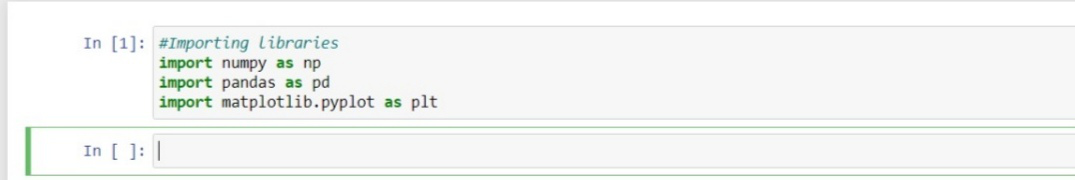### 3. Importing Dataset

Now, it’s time to import the dataset which we have collected for the Machine Learning Model. Before importing a dataset make sure to set the current directory as the working directory. Now to import the dataset use read_csv() of the pandas’ library which is used to read a CSV file and perform various operations on it. There are various other options available to read the file in different formats like read_excel() for reading excel files.

## Python

 `data ``=` `pd.read_csv(``'dataset.csv'``)`

The dataset is the name of the variable which stores the loaded dataset as filed named as a dataset in CSV format. Here is an example with a random dataset known as the Titanic dataset which you can download here.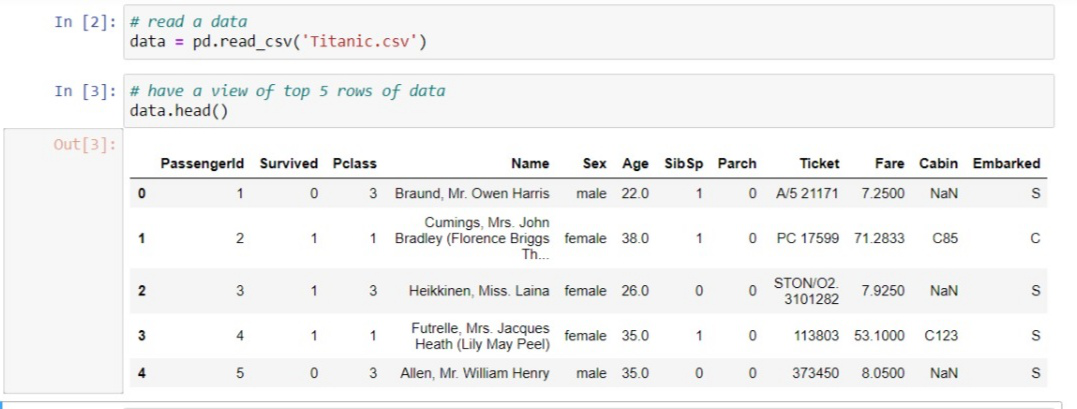Extracting Dependent And Independent Variables: With a filtered data set explored, you need to create a matrix of Independent variables and a vector of dependent variables. At first, you should decide and confirm that which columns or factors you are using as independent variables(also known as features) to train your model which affects your target variables. For example, If the target column is the last column of your dataset then you can easily create a matrix of your Independent variables as:

## Python

 `x ``=` `data.iloc[:,:``-``1``].values`

Here, the first colon (:) represents that we want all the lines in our dataset. :-1 means we want to take all the columns except the last one. And .values means we want to take all the values. And if the target variable is not at last as in our case (Survived) then we can drop that and take the all other columns in the train set. Example: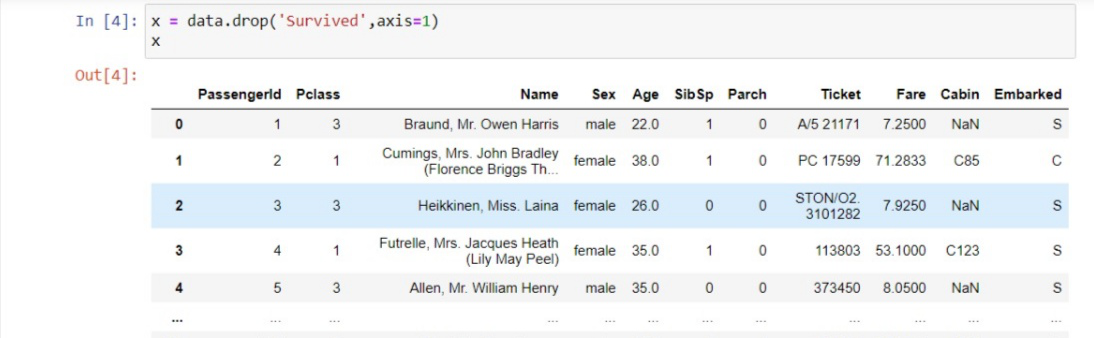And to take the vector of the dependent variable which is simply the last column of the dataset you can write as:

## Python

 `y ``=` `data.iloc[:,``-``1``].values`

Or use this method,

## Python

 `y ``=` `data[``'Survived'``]`

### 4. Dealing with missing values

Missing data can create huge problems to the results hence they are necessary to be removed from the dataset. Missing data is the most common problem and an important step involved in data cleaning. Missing values usually take the form of NaN or NONE. The cause of missing value is: sometimes most of the fields in columns are empty which needs to be filled by correct data and sometimes there is incorrect data or poor quality of data which adversely affects the outputs. There are several ways to deal with missing values and fill them:

• The first way to deal with NULL values: you can simply delete the rows or columns which are having NULL values. But most of the time this may lead to loss of information so this method is not so efficient.
• Another important method is to fill the data in place of NULL values. You can calculate the mean of a specific row and column and fill that in place of Null values. The method is very useful in which columns have numerical data such as age, salary, weight, year, etc.
• You can also decide to fill up missing values with whatever value comes directly after it in the same column.

The decisions depend on the type of data, and the results which you want to draw with the data. If missing values are less then it’s good to delete them and if more than 50% of values are missing then it needs to fill with correct data.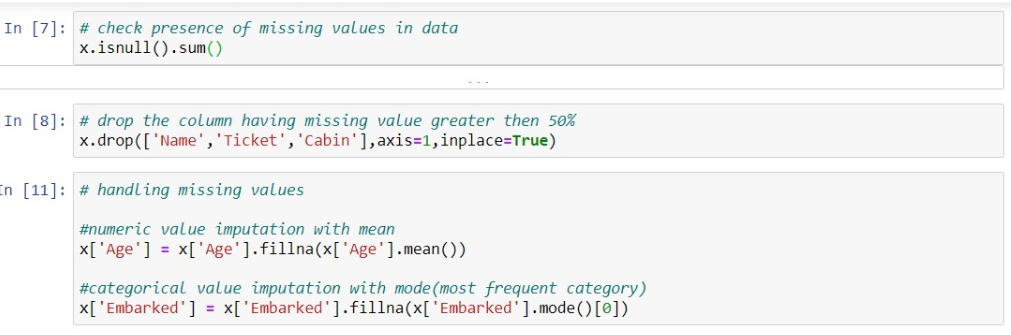Here, the cabin column contains approximately 55% missing data. name and ticket column are not so efficient to predict the person’s survival rate so drop the column which is not significant in prediction otherwise they will harm your machine learning model accuracy.

### 5. Encoding Categorical Data

Machine learning works with Mathematics and numbers(float or int type). However, it often happens that there are some columns in the dataset which have categorical values(string type) and we want to use them to train our model. In that case, we have to convert categories values to numeric types. For example, there is a sex column in a dataset containing values Male and Female which need to convert to numeric then we can convert it simply like we can assign 0 to Male and 1 to Female and train our model on it. It mostly happens with many columns such as the name of the State, Country, Color, etc. To convert values in numeric we can simply use SKLEARN preprocessing library which has the class name LabelEncoder(). For example, we take the state column and convert it to numeric values.

## Python

 `# CATEGORICAL DATA``from` `sklearn.preprocessing ``import` `LabelEncoder``le_x ``=` `LabelEncoder()``x[``'State'``] ``=` `le_x.fit_transform(x[``'State'``])`

In the above code, we have imported LabelEncoder class of preprocessing library from sklearn. The class has successfully encoded the variables in digits. If there are more digits encoded such as 0,1,2 then the machine learning model will assume there is some relationship between this And by these, the outputs predicted by the model will be wrong and performance will be reduced. So, to avoid this problem we will use dummy variables.

Dummy Variables: Dummy variables are the variables which have value only 0 and 1. In our dataset, if we have 3 values as 0,1 & 2 then it will convert it into 0 and 1. For this, we will use OneHotEncoder() class of preprocessing library. it is used when categories are less in a column such as a gender column has values as male and female then it can be encoded as 0,1.

## Python

 `from` `sklearn.preprocessing ``import` `OneHotEncoder``onehot_encoder ``=` `OneHotEncoder(categorical_features ``=` `[``'State'``])``x ``=` `onehot_encoder.fit_transform(x).toarray()` `# or``x ``=` `pd.get_dummies(x[``'gender'``])`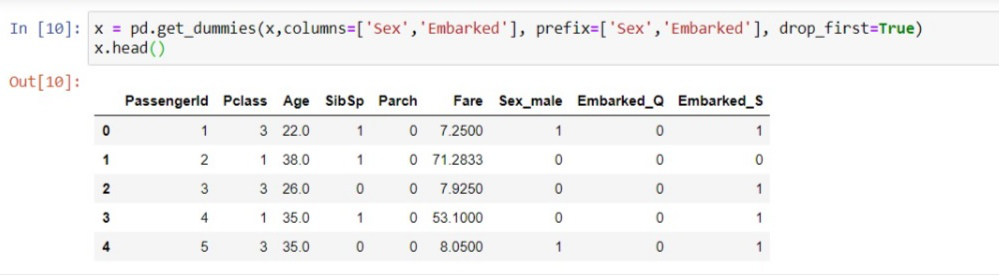### 6. Splitting dataset into training and testing set

The very crucial step of data preprocessing to split the dataset into training and testing sets. By doing this step we can enhance the performance and accuracy of our model in a better and efficient way. If we train the model on the different datasets and test it with a completely different dataset from training then it will create difficulties for the model to estimate the creation between independent and dependent variables and the accuracy of the model will be very less. So, we always try to make a machine learning model that performs well with the training set as well with the testing set.

• Training set: Subset of the dataset to train the model, the outputs are known to us as well to model.
• Testing set: Subset of the dataset to test the model, which model predicts the output based on training given to the model.

For this, we use the train_test_split class of model_selection library from the sklearn.

## Python

 `from` `sklearn.model_selection ``import` `train_test_split``x_train, x_test, y_train, y_test ``=` `train_test_split(x, y,``                                                    ``test_size ``=` `0.2``,``                                                    ``random_state ``=` `0``)`

• x_train: independent variables(features) for training set
• y_train: features for testing data
• x_test: Dependent variables training data
• y_test: Independent variables testing data

In train_test_split() we passed 4 parameter in which two are arrays and the third one is test_size which define the size of testing data. Here we have taken 20% data as testing so given as 0.2 and the remaining 80% will be given to the training set. The last parameter random_state is used to set a seed for a random generator so that you always get the same result.

### 7. Feature Scaling

The final step of the machine learning model is feature scaling. It’s a method to Standardize the training dataset in a specific range. In feature scaling, all the values are kept in the same range and on the same scale so that no variable dominates the other variable. For example, we have age and salary in the training dataset then they are not on the same scale as age is 31 and salary is 48000. As Machine learning model is based on Euclidean Distance and if we did not scale the variable it will cause a problem in results and performance.

EUCLIDEAN DISTANCE BETWEEN A & B = ∑[(x2 – x1)² + (y2 – y1)²]

For feature scaling, we use the StandardScaler class from the preprocessing library.

## Python

 `from` `sklearn.preprocessing ``import` `StandardScaler`

Now, we will create an object of StandardScaler class for independent variables. And then we will fit and transform the training dataset. For the testing dataset, we will directly apply the transform function because it has already done in the training dataset.

## Python

 `std_x ``=` `StandardScaler()``x_train ``=` `std_x.fit_transform(x_train)``x_test ``=` `std_x.transform(x_test)`

As the output, the variables are scaled between -1 to 1. After performing these steps fit the model, predict and deploy it, and then test the accuracy of the model. It will give exact results and the accuracy of the model will always be above 90 percent.

### Combining All the Above Steps

Now, in the end, we will combine all the steps to make the code more readable and understandable.

## Python

 `# Importing libraries``import` `numpy as np``import` `pandas as pd``import` `matplotlib.pyplot as plt` `# import dataset``data ``=` `pd.read_csv(``'datasets/Titanic.csv'``)` `# extract dependent and independent features``x ``=` `data.drop(``'Survived'``, axis ``=` `1``)``y ``=` `data[``'Survived'``]` `# Missing value Imputation``# drop the unrelaven features``x.drop([``'Name'``, ``'Ticket'``, ``'Cabin'``],``       ``axis ``=` `1``, inplace ``=` `True``)` `# numeric value imputation with mean``x[``'Age'``] ``=` `x[``'Age'``].fillna(x[``'Age'``].mean())  ` `# categorical value imputation with mode(most frequent category)``x[``'Embarked'``] ``=` `x[``'Embarked'``].fillna(x[``'Embarked'``].mode()[``0``])` `# category encoding``x ``=` `pd.get_dummies(x, columns ``=` `[``'Sex'``, ``'Embarked'``],``                   ``prefix ``=` `[``'Sex'``, ``'Embarked'``],``                   ``drop_first ``=` `True``)` `# train-test split``from` `sklearn.model_selection ``import` `train_test_split``x_train, x_test, y_train, y_test ``=` `train_test_split(x, y,``                                                    ``test_size ``=` `0.2``,``                                                    ``random_state ``=` `0``)` `# feature scaling``from` `sklearn.preprocessing ``import` `StandardScaler``std_x ``=` `StandardScaler()``x_train ``=` `std_x.fit_transform(x_train)``x_test ``=` `std_x.transform(x_test)`

In the above code, we have covered the necessary steps required to prepare your data or for data preprocessing. But there are some of the areas and the datasets in which all the steps are necessary and in those cases, we can exclude them from our code.

My Personal Notes arrow_drop_up